Reach Us+44-1522-440391
A Simple Method for Sensitivity Analysis of Unmeasured Confounding | OMICS International
Journal of Biometrics & Biostatistics

Like us on:

# A Simple Method for Sensitivity Analysis of Unmeasured Confounding

Yasutaka Chiba*

Division of Biostatistics, Clinical Research Center, Kinki University School of Medicine, Japan

*Corresponding Author:
Yasutaka Chiba
Division of Biostatistics
Clinical Research Center
Kinki University School of Medicine
377-2, Ohno-higashi, Osakasayama
Osaka 589-8511, Japan
Tel: +81-72-366-0221
Fax: +81-72-368-1193
E-mail: [email protected]

Received date: August 09, 2012; Accepted date: August 10, 2012; Published date: August 15, 2012

Citation:Chiba Y (2012) A Simple Method for Sensitivity Analysis of Unmeasured Confounding. J Biom Biostat 3:e113. doi: 10.4172/2155-6180.1000e113

Copyright: © 2012 Chiba Y. This is an open-access article distributed under the terms of the Creative Commons Attribution License, which permits unrestricted use, distribution, and reproduction in any medium, provided the original author and source are credited.

Visit for more related articles at Journal of Biometrics & Biostatistics

#### Abstract

Unmeasured confounding is widely recognized as one of the principal problems faced by investigators conducting observational studies. Several sensitivity analysis techniques have been developed to handle unmeasured confounding [1-5]. Recently, VanderWeele and Arah  provided a general class of formulas for sensitivity analysis of unmeasured confounding. Their formulas benefit from the fact that they do not presuppose that any particular method is used to yield the initial estimate adjusted only for measured confounders. Three major methods to yield such an initial estimate are reviewed in the appendix. In this editorial, we describe a simple sensitivity analysis method that retains the advantages described above. The method has further advantages that there is only one sensitivity parameter and therefore the results can easily be displayed graphically, and computer programs to yield the initial estimate adjusted only for the measured confounders can be used for the sensitivity analysis without additional programming.

We use the following notation. Let A denote the exposure status of a particular individual. Suppose that A is dichotomous (A = 1 if exposed and A = 0 if unexposed). Let Y be the observed outcome of that individual. Let X denote a measured confounder or a set of measured confounders, and U denote an unmeasured confounder or a set of unmeasured confounders. We also consider the potential outcomes (or counterfactual) framework . Let Ya denote the potential outcome of Y for an individual if the exposure A, perhaps contrary to fact, had been set to value a. Using this notation, the causal effects with the total, exposed (A = 1), and unexposed (A = 0) groups as the target populations are provided by a comparison between E(Y1) and E(Y0), between E(Y1 | A = 1) and E(Y0 | A = 1), and between E(Y1 | A = 0) and E(Y0 | A = 0), respectively.

We assume that the potential outcome Ya for an individual does not depend on the exposure status of other individuals. This assumption is sometimes referred to as the no-interference assumption . Furthermore, we require the consistency assumption YA = Y, i.e., the value of Y that would have been observed if A had been set to its actual value is equal to the actually observed value of Y. Therefore, the only potential outcome for an individual that we observe is the potential outcome YA, i.e., the value of Y that would have been observed if A was set to its actual value. Finally, we suppose that the effect of A on Y is unconfounded given both X and U; in a counterfactual notation, Ya is independent of A conditional on X and U.

To propose sensitivity analysis formulas of unmeasured confounding for difference measures, we apply the sensitivity parameter introduced by Brumback et al. , originally presented in the context of the inverse probability weighting approach . This is represented by the following formula:

δa ≡ E(Ya | A = 1, X = x) – E(Ya | A = 0, X = x)

where it is assumed that the value of δa does not vary between the strata of X. When δa > 0, E(Ya | A = 1, X = x) > E(Ya | A = 0, X = x), meaning that the individuals in the exposed group tend to have larger values than those in the unexposed group in the stratum with x. Conversely, when δa < 0, E(Ya | A = 1, X = x) < E(Ya | A = 0, X = x), meaning that the individuals in the exposed group tend to have smaller values than those in the unexposed group in the stratum with x. There is no confounding when δa = 0.

Let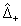,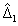, and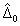denote the average outcome differences adjusted only for X when the target populations are the total, exposed, and unexposed groups, respectively. Then, using the sensitivity parameter δa, the causal effects for the difference measures can be expressed as follows: For the causal effect with the total group as the target population,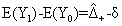where δ = δ1Pr(A = 0) + δ0Pr(A = 1) is a weighted mean of δ0 and δ1; for the exposed group,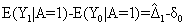and for the unexposed group,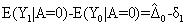Note that δ takes a value between δ0 and δ1.

These sensitivity analysis formulas indicate that the causal effects for the difference measures can simply be expressed as the difference between the initial estimate and a sensitivity parameter, and thus we can easily conduct a sensitivity analysis. The sensitivity parameter δ (δ0 or δ1) is set by the investigator according to what is thought to be plausible. The parameter can be varied over a range of plausible values to examine how conclusions vary according to different parameter values. To obtain the confidence intervals of the true causal effect for the fixed values of δ (δ0 or δ1), δ (δ0 or δ1) can be simply subtracted from the upper and lower confidence limits for the average outcome difference. Therefore, we can readily display the results of sensitivity analysis graphically, where the horizontal line represents the sensitivity parameter and the vertical line represents the true causal effect. However, for the total group as the target population, because δ depends on Pr(A = a), strictly, the variance of this probability should be taken into account to obtain the confidence intervals.

If we are sure that the individuals in the exposed group tend to have larger values than those in the unexposed group in the stratum with x, i.e., E(Ya | A = 1, X = x) ≥ E(Ya | A = 0, X = x), it would be assumed that δa ≥ 0. Conversely, if we are sure that E(Ya | A = 1, X = x) ≤ E(Ya | A = 0, X = x), it would be assumed that δa ≤ 0. Note that E(Ya | A = 1, X = x) ≥ E(Ya | A = 0, X = x) holds when both relationships between the unmeasured confounder-outcome and the unmeasured confounder-exposure are positive or negative in the stratum with x [9,10]. The reverse results are obtained when one of the relationships is positive and the other is negative. The other assumptions to derive a range of the sensitivity parameter are found elsewhere .

The above sensitivity analysis formulas for difference measures can be straightforwardly extended to ratio measures. Here, we assume that the outcome is binary; i.e., E(Y | A = a) = Pr(Y = 1 | A = a) and E(Ya) = Pr(Ya = 1). To propose the sensitivity analysis formulas for ratio measures, we introduce the following sensitivity parameter  instead of δa: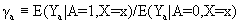where it is assumed that the value of γa, similar to δa, does not vary between the strata of X. Whether the value of γa is greater or less than 1 is interpreted in a similar manner to whether, the value of δa is larger or smaller than 0.

Let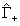,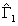, and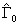denote the average outcome ratios adjusted only for X when the target populations are the total, exposed, and unexposed groups, respectively. Then, using the sensitivity parameter γa, the causal effects for the ratio measures can be expressed as the ratio between the initial estimate and a sensitivity parameter. The formulas are follows: For the causal effect with the total group as the target population,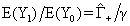where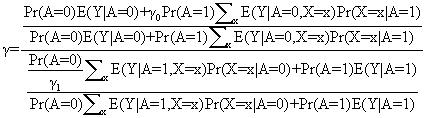(1)

for the exposed group,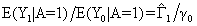and for the unexposed group,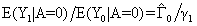While the sensitivity analysis formulas for the exposed and unexposed groups are simple, the formula for the total group is complex and causes problems for the interpretation. However, when both γ0 and γ1 are greater (less) than 1, γ is also greater (less) than 1 and takes a value between the values of γ0 and γ1.

A sensitivity analysis for ratio measures can also be conducted easily, and the procedure is identical to that for difference measures. Using the log scale to obtain the confidence intervals of the true causal effect, a sensitivity parameter can be simply subtracted from the upper and lower confidence limits for the initial estimate. However, for the total group as the target population, strictly, the variances of estimators in (1) should be taken into account and it is troublesome to obtain the exact confidence intervals.

In this editorial, we have described a simple method for sensitivity analysis of unmeasured confounding in three target populations: total, exposed, and unexposed groups. The method can also be applied to the attributable fraction . While the method described here has advantages mentioned at the beginning, it has a disadvantage that we need a strong assumption that the values of δa and γa do not vary between the strata of X. This assumption may not be reasonable in many actual studies. However, it is troublesome to set each value of the sensitivity parameters within each stratum of X, and further somewhat complex programming will be required to conduct a sensitivity analysis. In addition, the result of a sensitivity analysis with this assumption may not be largely different from that without the assumption, although the former always has the narrower confidence intervals than the latter.

Sensitivity analysis will aid in exploration of the potential impact of unmeasured confounding. We recommend performing a sensitivity analysis to evaluate the influence of unmeasured confounders on study results.

Appendix: Adjustment for Measured Confounding

We here introduce three approaches to adjust for the measured confounders: the model-based standardization approach, the Inverse Probability Weighting (IPW) approach, and the Doubly Robust (DR) estimation. The estimators from these three approaches are summarized in Table 1. In this table, i = 1, …, n denotes an individual and n is the total number; n1 and n0 are the number of individuals in the exposed and unexposed groups, respectively. E(Y | A = 1) and E(Y | A = 0) are the average outcomes for individuals in the exposed and unexposed groups, respectively.

 Target population Average potential outcome Approach Model-based standardization Inverse probability weighting Doubly robust estimation Total E(Y1)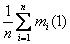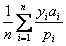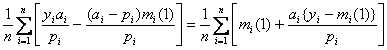E(Y0)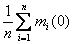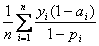Exposed E(Y1 | A = 1)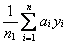E(Y0 | A = 1)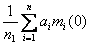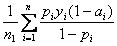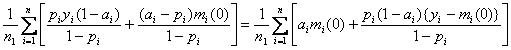Unexposed E(Y1 | A = 0)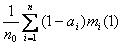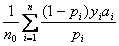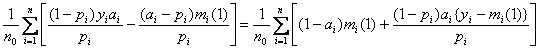E(Y0 | A = 0)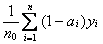Table 1: The estimators from the model-based standardization approach, inverse probability weighting approach, and doubly robust estimation.

The model-based standardization approach specifies a single model in which we simultaneously estimate the exposure-outcome association and the confounder-outcome association as follows:

where (α0, …, αk+1) is a set of regression parameters and can be estimated using standard software. Using this regression model, the expectations of the potential outcomes can be estimated as shown in Table 1, where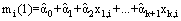is the predicted outcome given A = 1 for an individual i and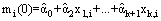is the predicted outcome given A = 0 for the individual i.

Rather than adjusting for the association between confounders and the outcome, we can control for confounding using the propensity score, which is defined as the conditional probability of exposure given confounders . The propensity score is typically estimated from the observed data with a model such as the following: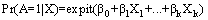where expit(s) = exp(s)/{1 + exp(s)}, and where (β0, …, βk) is a set of regression parameters that can be estimated by standard software. Using this regression model, the IPW approach estimates the expectations of the potential outcomes as in Table 1, where pi =expit(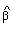+1X1,i + …, +kXk,i). Note that Sato and Matsuyama  exemplified an SAS code to yield the IPW estimate using the marginal structural model.

The DR estimation requires specification of two regression models for the outcome and exposure as a function of confounders. Having estimated mi(a) and pi, we combine these values as in Table 1 to calculate the DR estimates. These expressions suggest an intuitive explanation of the properties of DR. The formulas of the left hand side indicate that the DR estimators are equivalent to the unbiased estimators from the IPW approach if the exposure regression model is correctly specified. Likewise, the formulas of the right hand side indicate that the DR estimators are equivalent to the unbiased estimators from the modelbased standardization approach if the outcome regression model is correctly specified. Note that Funk et al.  presented an SAS macro to yield the DR estimate with the total group as the target population.

#### Acknowledgements

This work was supported partially by Grant-in-Aid for Scientific Research (No. 23700344) from the Ministry of Education, Culture, Sports, Science, and Technology of Japan.

#### References

Select your language of interest to view the total content in your interested language

### Article Usage

• Total views: 12255
• [From(publication date):
October-2012 - Mar 26, 2019]
• Breakdown by view type
• HTML page views : 8436

## Post your commentCan't read the image? click here to refresh
###### Peer Reviewed Journals

Make the best use of Scientific Research and information from our 700 + peer reviewed, Open Access Journals

International Conferences 2019-20

Meet Inspiring Speakers and Experts at our 3000+ Global Annual Meetings

Top